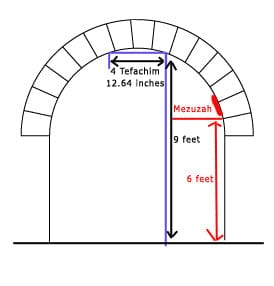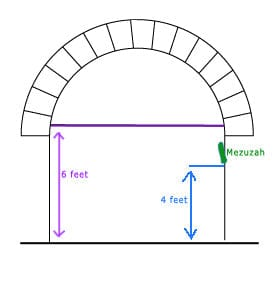# Mezuzah on an ArchwayPeople frequently ask, "How high up do I put the Mezuzah on an arch?" This is a bit of a complicated question. First let's make sure we understand the question. A regular door is rectangular in shape. The Halacha requires that the Mezuzah be placed at the 'bottom of the top third' of the door.  This means that you measure the door, divide by three and place the Mezuzah just above the one-third-from-the-top line.  You can read more about this here.

A problem arises with making this calculation with an arch.  What do we consider to be the top of the arch?  The most obvious answer would be to say that the highest point of the arch is the top and measure from there.  The problem is that according to Halacha, a door has to be at least 4 Tefachim-hand-breadths wide in order for it to be obligated in a Mezuzah.  At the highest point of an arch, it has not width at all because it is a single point on a curve.Because of this, we never measure the height of an arch from the highest point.  How then do we arrive at the proper calculation of where to put the Mezuzah?  There are two opinions.  The most widely accepted opinion is that we measure from the first place that you can square out 4 Tefachim.  (The most commonly accepted estimation of a tefach is 3.16 inches.)  This is the first place that the door is wide enough to be considered a door and therefore this is the top of the door.  We measure down from that point to the ground and divide by 3 to arrive at the height where the Mezuzah should be.  This is the most commonly accepted opinion.According to some others, including Chabad, we use a different calculation based on the Rambam.  The Rambam rules in the case of an arch that we consider the the place where the arch begins to curve as the top of the door.  This is as long as the straight part of the arch is at least 10 Tefachim high.  10 Tefachim being the minimum hight that a door needs to be in order to be obligated in a Mezuzah.  According to this opinion, we measure from the floor to the place where the curve starts.  We divide this by three and put the Mezuzah at the 'bottom of the top third' of the straight area.  In theory you could have an arch where the straight part is 3 feet high and the round part is 5 feet high.   According to this opinion, you would put the Mezuzah a bit more that 2 feet from the ground.  This would mean that the Mezuzah would be about knee hight.